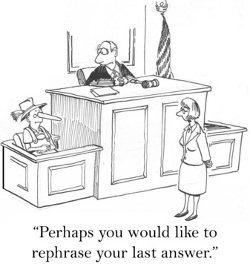# EquationsTo score 700+ on the GMAT, test takers must develop a strategy to answer the Quant questions in 2 minutes and Verbal questions in 1 minute & 20 seconds. Data sufficiency questions can be solved well within the 2-minute mark, most likely in 1 minute and 30 seconds if the conditions and question are rephrased. Not all questions will be required to be rephrased but there are certain conditions where this technique is extremely useful, especially when concepts in Ratio & Proportion, Equations, Inequalities & Divisibility are tested.EquationsThis is the most common question type where rephrasing the conditions might be useful, especially in quadratic equations, and questions that require finding roots. Let us look into a quadratic equation question typeFor the following equations with positive roots, the value of k is greater than one1) 3x^2 +5x + 2k = 02) (x+1)(3x+2) + 2k - 2 = 01) Statement (1) ALONE is sufficient, but statement (2) alone is not sufficient to answer the question asked.2) Statement (2) ALONE is sufficient, but statement (1) alone is not sufficient to answer the question asked.3) BOTH... Categories : Equations, Problem Solving In order to solve such equations, you need at least 2 distinct equations involving these unknowns.For example, if we are trying to solve for x and y, we won't be able to solve it using these 2 equations.2x + y = 144x + y - 14 = 14 - yWhy?  Because the two equations on top are the same.  If you simplify the second equation, you get 4x + 2y = 28 which reduces to 2x + y = 14 - the same equation as the first.  If the two equations are the same, then there will be infinitely many values for x and y that will satisfy the equations.  For example, x = 2 and y = 10 satisfies the equation.  So does x = 4 and y = 8.  And so does x = 6 and y = 2.In order to solve for an actual value of x and y, we need 2 distinct equations.For example, if we had2x + y = 14 --------(1)x - y = 4 ----------(2)Then from equation (2), we can get x = 4 + y and substitute that into equation (1) to get:2(4 + y) + y = 14  We can then solve for y.  See if you got y = 2  Once you've got y = 2, you can substitute that into x= 4 + y to get x = 6.An important lesson here is that you need as many distinct equations... Categories : Number Properties, Equations, Fractions If Y < 0 and -1 < -1 + X/Y < 0, which must be true? 1. X < 0 2. Y/X>1 3. Y^2-X^2 > 0 A. I only B. II only C. I and II only D. II and III only E. I, II, and III Answer If X is negative, and we already know that Y is negative, than X/Y would have to be positive (a negative divided by a negative is always positive). This means that for all values in which Y is smaller than X, X/Y would be between 0 and 1. More importantly, X must be negative, because if it were positive, the fraction X/Y would be negative. Statement I must be true. Statement II claims that Y/X>1. We already know that both X and Y are negative. We also know that Y has a larger absolute value. Statement II must also be true. On statement III, since we're talking about numbers squared, we can forget about the signs. We only need to figure out which of the two variables is greater. Statement III is true as well. Correct Answer - Choice E

Get F1GMAT's Newsletters (Best in the Industry)

• Ranking Analysis
• Post-MBA Salary Trends
• Post-MBA Job Function & Industry Analysis
• Post-MBA City Review
• MBA Application Essay Tips
• School Specific Essay Tips
• GMAT Preparation Tips